# Algebra II : Solving Radical Equations

## Example Questions

1 2 3 4 5 6 7 8 9 10 12 Next →

### Example Question #111 : Solving And Graphing Radicals

If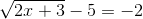, then?

-3

-4

4

3

1

3

Explanation:

We will begin by adding 5 to both sides of the equation.Next, let's square both sides to eliminate the radical.Finally, we can solve this like a simple two-step equation. Subtract 3 from both sides of the equation.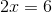Now, divide each side by 2.Finally, check the solution to make sure that it results in a true statement.### Example Question #112 : Solving And Graphing Radicals

Solve for: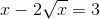ororExplanation:

When working with radicals, a helpful step is to square both sides of an equation so that you can remove the radical sign and deal with a more classic linear or quadratic equation. But of course if you were to simply square both sides first here, you would still end up with radical signs, as were you to FOIL the left side you wouldn't eliminate the radicals.  So a good first step is to add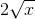and subtract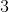from both sides so that you get: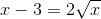Now when you square both sides, you'll eliminate the radical on the right-hand side and yield: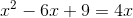Then when you subtract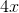from both sides to set up a quadratic equalling zero, you have a factorable quadratic: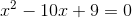This factors to: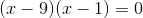Which would seem to yield solutions of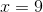and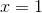.  However, when you're solving for quadratics it's always important to plug your solutions back into the original equation to check for extraneous solutions.  Here if you plug inthe math holds: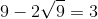because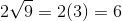, and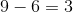.

But if you plug in, you'll see that the original equation is not satisfied: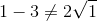because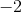does not equal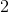.  Therefore the only proper answer is.

### Example Question #113 : Solving And Graphing Radicals

Solve for: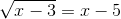No solution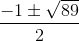ororororExplanation:

To solve, first square both sides: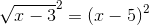squaring the left side just givs x - 3. To square the left side, use the distributite property and multiply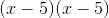: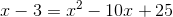This is a quadratic, we just need to combine like terms and get it equal to 0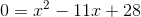now we can solve using the quadratic formula: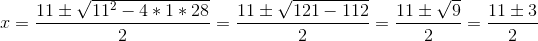This gives us 2 potential answers: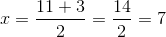and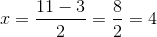1 2 3 4 5 6 7 8 9 10 12 Next →

### All Algebra II Resources